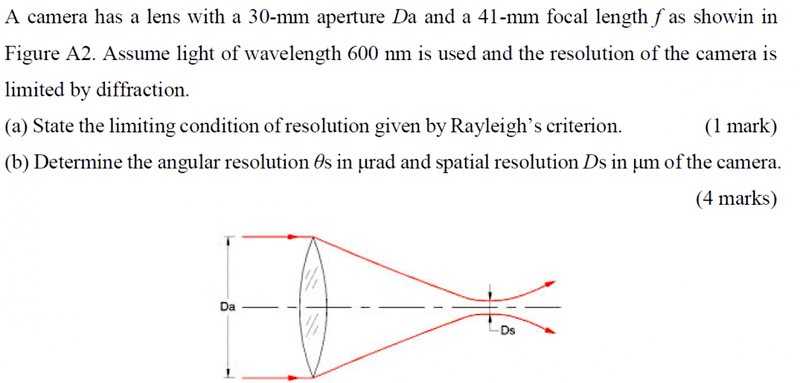• kenok1216

## Homework Statementθmin=1.22λ/D

## The Attempt at a Solution

(a)θmin=1.22λ/D
(b)θs=1.22(600nm)/30mm=24.4μm but what is spatial resolution and how to calculate it??

## Homework Statement

View attachment 99604

θmin=1.22λ/D

## The Attempt at a Solution

(a)θmin=1.22λ/D
(b)θs=1.22(600nm)/30mm=24.4μm but what is spatial resolution and how to calculate it??
That answer should be in units appropriate for an angle, certainly not μm .

what is spatial resolution and how to calculate it??
It's related to the angular resolution. You can think of the spatial resolution as the arc length of a circle subtended by the angle of angular resolution.

That answer should be in units appropriate for an angle, certainly not μm .

Yes.

It's related to the angular resolution. You can think of the spatial resolution as the arc length of a circle subtended by the angle of angular resolution.
s=rθ, so what is r is this question?object distance? focal length?image distance?

s=rθ, so what is r is this question?object distance? focal length?image distance?
About the circle I was talking about, you can assume it to be centered at the center of the lens and since your image is located at the focal plane, the arc length of interest must touch the focal plane. What will r be?

About the circle I was talking about, you can assume it to be centered at the center of the lens and since your image is located at the focal plane, the arc length of interest must touch the focal plane. What will r be?
Focal lenght?

Focal lenght?
Yes.

Yes.
Thank